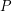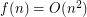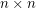# Universal point sets for planar graphs

 Importance: High ✭✭✭
 Author(s): Mohar, Bojan
 Subject: Graph Theory » Topological Graph Theory » » Drawings
 Keywords: geometric graph planar graph universal set
 Posted by: mdevos on: May 22nd, 2007

We say that a setis-universal if everyvertex planar graph can be drawn in the plane so that each vertex maps to a distinct point in, and all edges are (non-intersecting) straight line segments.

Question   Does there exist an-universal set of size?

More generally, if we letdenote the size of the smallest-universal set, we are interested in the behaviour of. The best known upper bound is. Indeed, every-vertex planar graph can be drawn as required in thegrid [dFPP], [S]. On the flip side, it is known thatfor sufficiently large[CH].

## Bibliography

[CH] M. Chrobak and H.Karloff. A lower bound on the size of universal sets for planar graphs. SIGACT News, 20:83-86, 1989.

[dFPP] H. de Fraysseix, J. Pach, and R. Pollack. How to draw a planar graph on a grid. Combinatorica, 10(1):41-51, 1990. MathSciNet

[S] W. Schnyder. Embedding planar graphs on the grid. In Proc. 1st ACM-SIAM Sympos. Discrete Algorithms, pages 138-148, 1990.

* indicates original appearance(s) of problem.